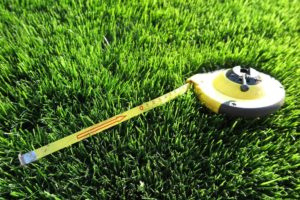# How to Measure the Area of Your Planned LawnThere’s nothing more satisfying than getting work done around your house. DIY is blowing up in the world, so why not DIY your front or back lawn? You’ll feel satisfaction every time you stroll up to your house of knowing that you were the one responsible for the hard work necessary to create a beautiful and healthy lawn.

But first you must prepare your site and soil for some new turf. You can read how to do that here.

### The importance of Measuring

It is incredibly important to be meticulous about measuring your space so that you order exactly the right square metres of turf. You’ll also need to keep in mind what other aspects of your lawn there will be. For example, if you are incorporating any gardens, pathways or pools, you’ll need to account for those in your calculations. It’s a good idea to get a pencil and a paper and draw a rough, but more or less to scale, sketch of what you plan your lawn and new turf to look like.

We at Glenview Turf in Sunshine Coast are here to help if you aren’t sure how to go about measuring your lawn. It all depends on the shapes, so check out the formulas below to calculate your space in square metres.

### Square or Rectangle

Measure the LENGTH and WIDTH of the Square or Rectangular area in metres. Then, multiply the length by the width to get the total measurement.

Example: If the Length is 10 metres, and the Width is 5à 10 x 5= 50 square metres.

### Circle or Oval

Measure the DIAMETER of the Circular area in metres. To calculate, multiply by 0.80 by the diameter and then by diameter again.

Example: If the Diameter is 9 metresà 0.80 x 9 x 9= 64.80 square metres.

### Triangle

Measure the BASE and HEIGHT of the Triangular area in metres. To calculate, multiply 0.50 by the base and then by the height.

Example: If the Base is 40 metres and the Height 25 metres à 0.50 x 40 x 25 = 500 square metres.

### Trapezoid

Measure in metres PARALLEL SIDES (1) and (2) and HEIGHT perpendicular to the Parallel Sides. Multiply 0.50 by the sum of the two parallel sides and then by the height to calculate the space.

Example: If Parallel Side (1) is 30 metres, Parallel Side (2) is 50 metres, and the Height is 20 metresà 0.50 x (30+50=80) x 20 = 800 square metres.

## Unusual Shapes

First, divide the area into sections of regular shapes, like circles, squares and triangles. Then measure those using the guidelines above and calculate by applying the formulas mentioned above.

### Different Widths

First measure the length of the longest axis across the area. Then, every two metres along the length line measure the width of the area at right angles to the length line. Total all widths, divide by number of width section and multiply by length.

Example: The Length is 9 metres, Width (1) is 3 metres, Width (2) is 5 metres and Width (3) is 4 metresà 3+5+4=12 divide by 3 = 4 x 9 = 36 square metres.

If you have any questions on how to measure your land for new turf, or would like a quote so a specialist can take care of it for you, please call Glenview Turf today.